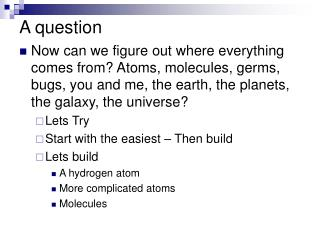DownloadDownload PresentationA question

# A question

Download Presentation## A question

- - - - - - - - - - - - - - - - - - - - - - - - - - - E N D - - - - - - - - - - - - - - - - - - - - - - - - - - -
##### Presentation Transcript

1. A question • Now can we figure out where everything comes from? Atoms, molecules, germs, bugs, you and me, the earth, the planets, the galaxy, the universe? • Lets Try • Start with the easiest – Then build • Lets build • A hydrogen atom • More complicated atoms • Molecules

2. Our tools Schrödinger (a 3D version) U(x)=Ze2/r A “classical” picture (for our human brains” Electrons orbiting around a nucleus with charge Z

3. Thinking “classically” • Electron going around nucleus of charge Z • Planet going around sun • Different planets have different orbits [n] • N=1 = mercury, earth might be n=3 • Planets have angular momentum [L] • Planet orbits have a tilt [mL] • Planets spin around their axis [S] • Planet spin has a tilt! [mS]

4. Use a 3-D version of this • U(x)=Ze2/r Rydberg’s Constant=R=13.6eV

5. Here it is: and its sort of like planets We Get 3 Quantum numbers! n ~ Energy level L ~ Orbital Angular momentum mL~ z-component of L Does the electron have spin??

6. Stern-Gerlach Experiment • A beam of neutral silver atoms is split into two components by a nonuniform magnetic field • The atoms experienced a force due to their magnetic moments • The beam had two distinct components in contrast to the classical prediction If the electron had spin, it might explain this

7. SZ SZ Intrinsic Spin Violates our intuition: How can an elementary particle such as the e¯ be point like, wavelike and have perpetual angular momentum? Electron Spins • Spin quantized • The electron can have spin S= • up • down • In the presence of a magnetic field, the energy of the electron is slightly different for the two spin directions and this produces doublets in spectra of certain gases ms = +1/2 or -1/2 SZ=ms

8. Electron Spins, cont • The concept of a spinning electron is conceptually useful • The electron is a point particle, without any spatial extent • Therefore the electron cannot be considered to be actually spinning • The experimental evidence supports the electron having some intrinsic angular momentum that can be described by ms • Sommerfeld and Dirac showed this results from the relativistic properties of the electron

9. Spin was first discovered in the context of the emission spectrum of alkali metals - "two-valued quantum degree of freedom" associated with the electron in the outermost shell. In trying to understand splitting patterns and separations of line spectra, the concept of spin appeared "it is indeed very clever but of course has nothing to do with reality". W. Pauli A year later Goudsmit and Uhlenbeck, published a paper on this same idea. Pauli finally formalized the theory of Spin in 1927

10. The Exclusion Principle • The four quantum numbers discussed so far can be used to describe all the electronic states of an atom regardless of the number of electrons in its structure • The Exclusion Principle states that no two electrons in an atom can ever be in the same quantum state • Therefore, no two electrons in the same atom can have the same set of quantum numbers

11. Orbitals • An orbital is defined as the atomic state characterized by the quantum numbers n, l and ml • From the Exclusion Principle, it can be seen that only two electrons can be present in any orbital • One electron will have ms = ½ and one will have ms = -½

12. The arrows represent spin The n=1 shell can accommodate only two electrons, since only one orbital is allowed In general, each shell can accommodate up to 2n2 electrons Allowed Quantum States, Example

13. Many-electron atoms The fact that two electrons could be in any energy level corresponding to a given set of quantum numbers (n,l,m) led eventually to the discovery of the spin of the electron. The energy levels corresponding to n = 1, 2, 3, … are called shells and each can hold 2n2 electrons. The shells are labeled K, L, M, … for n = 1, 2, 3, ….

14. Fig 42-19, p.1376

15. Model of H atom particle in a box E=-13.6 ev (n=1 state) top of the box be at zero width of the box is ~ 2aB~0.1 nm 2 H atoms for a bond Separation =.12 nm match solutions at boundary Covalent bonds Ge-κx outside solution Match solutions Asin(kx) inside solution

16. Now solve for the new energies n=1 n=2 E=-9 eV E=-17.5 eV prob density prob density

17. Total energy – the covalent bond • If the total energy is negative, then the particles are bound. • pp repulsive energy = • n=1 E=12 eV-17.5eV = -5.5eV • covalent bond!! • n=2 E=12 eV – 9 eV=+3eV • doesn’t bind

18. Lasers Excitation Simulated Emmision A laser Emmission

19. Lasers • CD • Telecommunications • Surgery • Etc etc Optical Tweezers, DNA making an R Fig 42-27, p.1386

20. If the exclusion principle were not valid, • a. every electron in an atom would have a different mass. • b. every atom would be in its state of greatest ionization. • c. the quantum numbers and would not exist. • d. every pair of electrons in a state would have to have opposite spins. • e. every electron in an atom would end up in the atom’s lowest energy state.